Next: Non-recursive implementation of predictive parsing Up: Parsing Previous: Top-down parsing

## Predictive parsing

THE IDEA. Predictive parsing relies on information about what first symbols can be generated by the right side of a production. The lookahead symbol guides the selection of the production A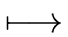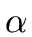to be used:
• ifstarts with a token, then the production can be used when the lookahead symbol matches this token
• ifstarts with a nonterminal B, then the production can be used if the lookahead symbol can be generated from B.

THE METHOD.

(1)
For each nonterminal A for each production Alet FIRST() be the subset of VT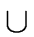{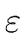} defined by the following rules
• for every a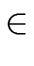VT we have

 a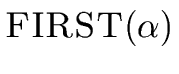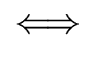(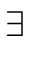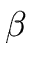(VT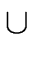VN) * )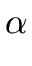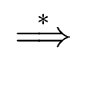a(40)

•FIRST(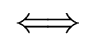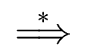In other words the token a belongs to FIRST() iff there exists a string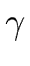deriving fromsuch that a is the first symbol of. Moreoverbelongs to FIRST() iffderives from.
(2)
Consider the following procedure in pseudo-code of Algorithm 1.

Algorithm 1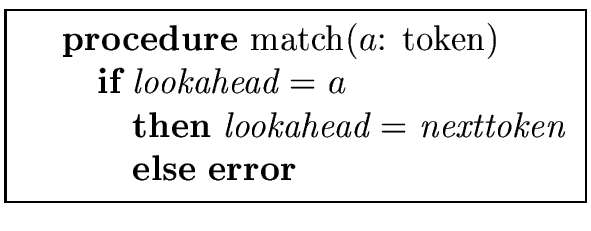So match(a) moves the cursor lookahead one symbol forward iff lookahead points to a. Otherwise an error is produced.
(3)
A procedure proc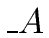(given by Algorithm 2) is associated with every nonterminal A.

Algorithm 2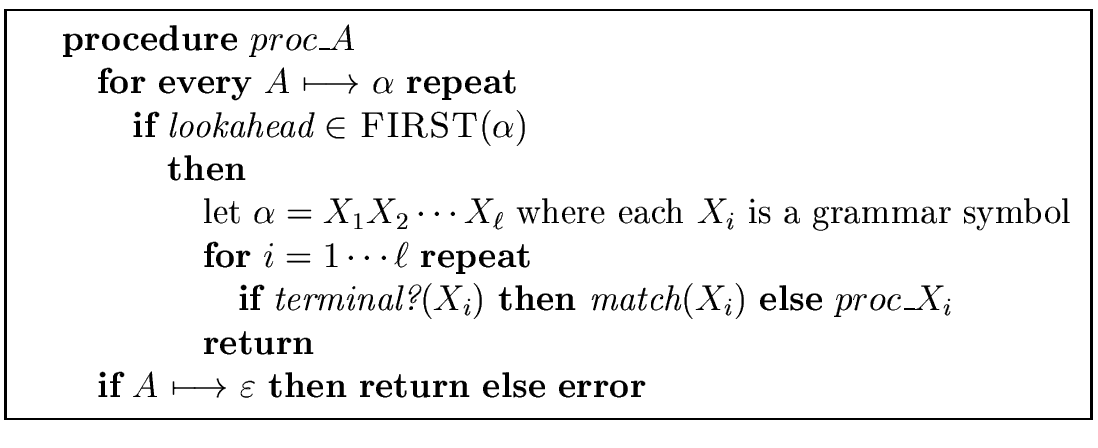In other words, given a nonterminal A the call procchooses an A-production Asuch that lookahead belomgs to FIRST(). If such a production exists then for each symbol X in(readingfrom left to right)
• if X is a terminal, then call match(X)
• if X is a nonterminal, then call proc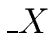If no A-production Asatisfies lookaheadFIRST() then either Ais a production and the procedure terminates normally, or an error is produced.
(4)
Initially lookahead is the first symol of the input string and we run procS where S is the start symbol.
Observations:
• In Algorithm 2 return is an escape statement without error whereas error is an interruption with error.
• The grammar must have no left recursive derivations, otherwise the parsing may lead to an infinite loop.
• Back-tracking is avoided provided that for every nonterminal A and for every string of symbols,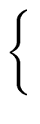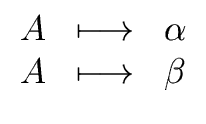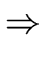FIRST()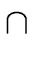FIRST()  =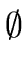. (41)

• The last statement of Algorithm 2 means that the A-production Acan be chosen if the lookahead symbol is not in any of the FIRST() for Awith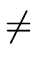.
Hint: You should understand the production Aas a way to say that the nonterminal A can be canceled (if no other A-production is convenient to use).

Example 15   Consider the following grammar (with terminals a, b, c, d and nonterminals S, A)
 ScAd Aab  | a
Here we have FIRST(cAd) = {c}, FIRST(ab) = {a} and FIRST(a) = {a}. Unfortunately, if we have lookahead = a we cannot tell each A-production to use. So left factoring would be needed to process this example further (what we will not do).

Example 16   The following grammar generates a subset of the types in the PASCAL language.
 typesimple |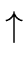id | array[simple] of type simpleinteger | char | num dotdot num
where
• all bold names and the characters,, [, ] are tokens,
• type and simple are nonterminals,
• dotdot stands for ...
• and num for a type of small integers.
Here's a string of tokens generated by the above grammar.
array [ num dotdot num ] of integer
We give now the FIRST sets of every left side of the above productions.
 FIRST(simple) = { integer, char, num } FIRST(id) = {} FIRST(array[simple] of type) = { array } FIRST(integer) = { integer } FIRST(char) = { char } FIRST(num dotdot num) = { num }
Algorithms 3 and 4 define the procedures proc_simple and proc_type.

Algorithm 3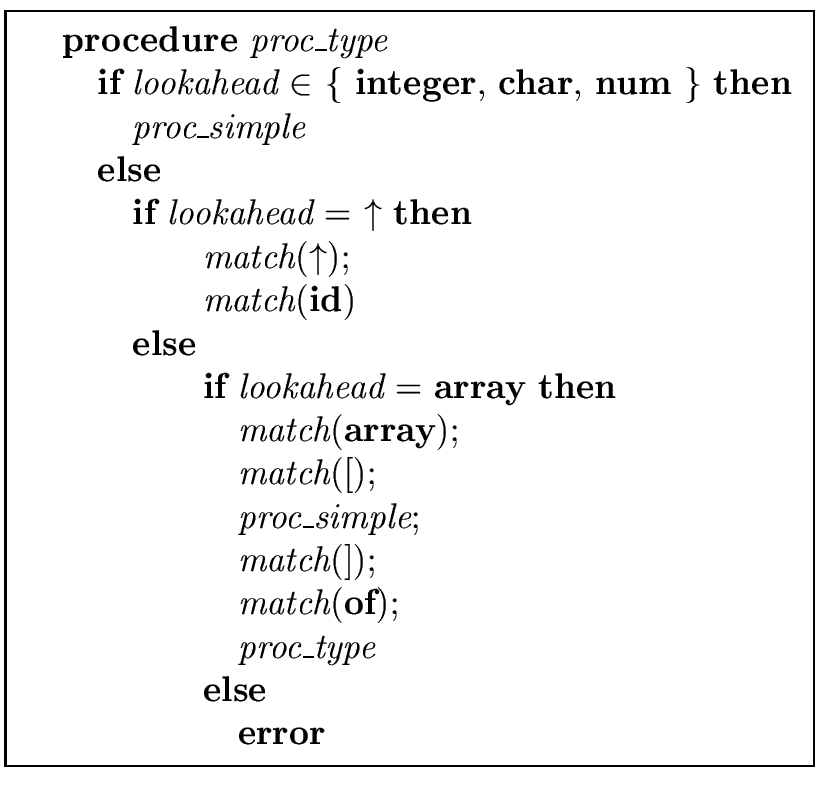Algorithm 4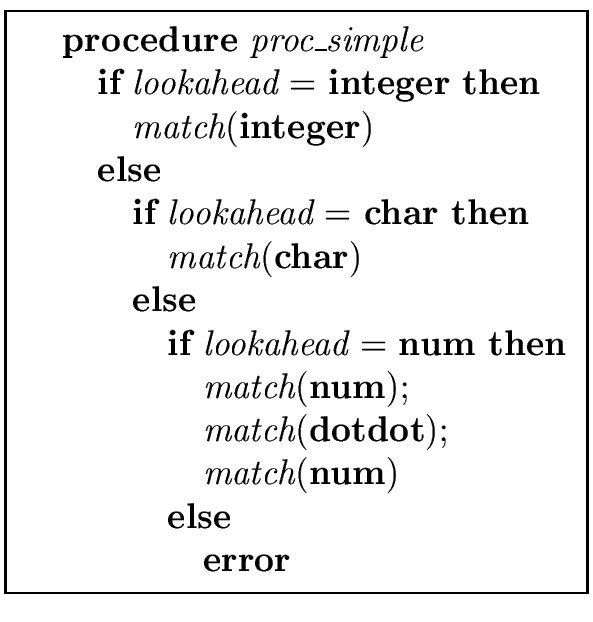Next: Non-recursive implementation of predictive parsing Up: Parsing Previous: Top-down parsing
Marc Moreno Maza
2004-12-02